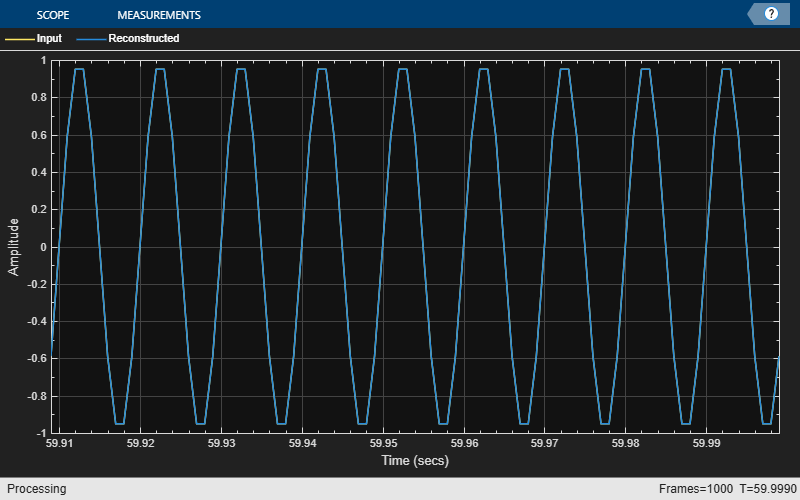# Transmit Complex Data over UDP Network

Compute the STFT of a sine wave and transmit the complex STFT data over a UDP network. At the receiver side, compute the ISTFT of the received data. Visualize the data sent and the data received using a time scope.

The `dsp.UDPSender` object can send complex data. In order to enable the `dsp.UDPReceiver` object to receive complex data, set the `IsMessageComplex` property to `true`.

```udps = dsp.UDPSender('RemoteIPPort',31000); udpr = dsp.UDPReceiver('LocalIPPort',31000,... 'IsMessageComplex',true,... 'MessageDataType','double'); setup(udpr); bytesSent = 0; bytesReceived = 0; dataLength = 128;```

Initialize the `dsp.STFT` and `dsp.ISTFT` System objects with a periodic `hann` window of length 120 samples and an overlap length of 60 samples. Set the FFT length to 128.

```winLen = 120; overlapLen = 60; frameLen = winLen-overlapLen; stf = dsp.STFT(... 'Window',hann(winLen,'periodic'),... 'OverlapLength',overlapLen,'FFTLength',128); istf = dsp.ISTFT(... 'Window',hann(winLen,'periodic'),... 'OverlapLength',overlapLen,... 'WeightedOverlapAdd',0);```

The input is a sinusoidal signal with a frequency of 100 Hz, a sample rate of 1000 Hz, and with 60 samples per each signal frame.

```sine = dsp.SineWave(... 'SamplesPerFrame',winLen-overlapLen,... 'Frequency',100);```

Initialize a `timescope` object with a sample rate of 1000 Hz and a time span of 0.09. The `Delay` object corrects the overlap length while comparing the input with the reconstructed output signal.

```ts = timescope('SampleRate',1000,... 'ShowLegend',true,... 'YLimits',[-1 1],... 'TimeSpanSource','Property',... 'TimeSpan',.09,... 'ChannelNames',{'Input','Reconstructed'}); dly = dsp.Delay('Length',overlapLen);```

Transmit complex STFT data of the sine wave over the UDP network. Due to the undependable nature of the UDP protocol, the data packets can get lost during transmission. The receiver is not guaranteed to receive all the data that you send.

Compute the ISTFT of the received data. Compare the input `x` to the reconstructed output `y`. Due to the latency introduced by the objects, the reconstructed output is shifted in time compared to the input. Therefore, to compare, take the norm of the difference between the reconstructed output `y` and the previous input, `xprev`.

Visualize the signals using a time scope. You can see that the reconstructed signal overlaps very closely with the input signal.

```n = zeros(1,1e3); xprev = 0; for k = 1:1e3 x = sine(); X = stf(x); bytesSent = bytesSent + length(X); udps(X); dataReceived = []; while (isempty(dataReceived)) dataReceived = udpr(); end if (~isempty(dataReceived)) y = istf(dataReceived); end n(1,k) = norm(y-xprev); xprev = x; bytesReceived = bytesReceived + length(dataReceived); ts([dly(x),y]); end```The norm of the difference is very small, indicating that the output signal is a perfectly reconstructed version of the input signal.

`max(abs(n))`
```ans = 5.3870e-14 ```

Release the UDP objects.

```release(udps); release(udpr);```

Some of the packets sent can be lost during transmission due to the lossy nature of the UDP protocol. To check for loss, compare the bytes sent to the bytes received.

`fprintf('Bytes sent: %d\n', bytesSent);`
```Bytes sent: 128000 ```
`fprintf('Bytes received: %d\n', bytesReceived);`
```Bytes received: 128000 ```